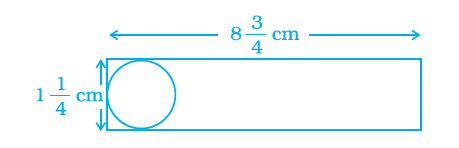# Shalini has to cut out circles of diameter 11/4 cm from an aluminum strip of dimensions 83/4 cm by 11/4 cm. How many full circles can Shalini cut? Also calculate the wastage of the aluminum strip.Given

Diameter of the circle = Breadth of the aluminium strip $1\frac{1}{4}$cm = $\frac{5}{4}$cm

Length of aluminium strip = $8\frac{3}{4}$cm = $\frac{35}{4}$cm

Find out

We have to determine the number of full circles can Shalini cut and also the wastage of the aluminum strip

Solution

∴The number of full circles cut from the aluminum strip = (35/4) ÷ (5/4)

= (35/4) × (4/5)

= (7/1) × (1/1)

= 7 circles

Radius of circle = (5/ (4 × 2)) = 5/8 cm

Area to be cut by one circle = πr2

= (22/7) × (5/8)2

= (22/7) × (25/64) cm2

Area to be cut by 7 full circles = 7 × (22/7) × (25/64)

= (22 × 25)/64

= 550/64 cm2

Area of the aluminum strip = length × breadth

= (35/4) × (5/4) cm2

= (175/16) cm2

∴The wastage of aluminum strip = (175/16) – (550/64)

= (700 – 550)/64

= 150/64

= 75/32 cm2

Number of circles sut by shalini= 7

The wastage of aluminum strip = 75/32 cm2(0)(0)